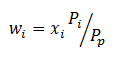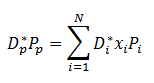# Sensitivity Measures for a Portfolio

In financial markets assets are managed at a portfolio level which in other words means a large number of securities are managed in a group. It would be very inconvenient to consider movements in each position that composes the portfolio individually. A more practical and efficient solution would be to measure and manage the sensitivities across the whole portfolio.

A practical example would help calrify how the relationships are used in the market. Let’s assume that the market expects rates to decrease in the future. Rates are inversely proportional to prices which means the duration would be expected the decrease. The portfolio manager would then adjust the duration of the portfolio upwards relative to the benchmark so that the effect of the rate decrease has a more pronounced effect on the duration of the portfolio as compared to the benchmark. This in turn means that the gains of the portfolio will outperform the gains of the benchmark given the future rate scenario.

The portfolio’s total market value is a sum of the market values of the components.  The portfolio weight is defined as follows:This assumes that the portfolio market value is not zero. The duration at the portfolio level is equal to the summation of the weights and the component durations and similarly the convexity at the portfolio level can be equated to the summation of the weights and the component convexities.

The portfolio modified duration can be represented by the equation below:Where the left side denotes the portfolio modified duration and value and Di* xi* Pi are the duration, number of units and values of each individual components of the portfolio.

A barbell portfolio is composed of instruments which have very long and short maturities and the contrast to that is the bullet portfolio which consists of instruments within the range. Due to the instrument with a very long maturity the barbell portfolio has a higher convexity compared to the bullet portfolio. For a large movement in yields this portfolio normally outperforms the bullet portfolio. Normally a barbell portfolio is structured to mirror the duration of a benchmark. In order to achieve the dserired effect an investment is made into securities with short and long maturities and a system of equations is solved to find out how the weights must be distributed between the component investments such that the duration of the barbell replicates the duration of the benchmark.

These measures at a portfolio level help managers determine how to actively manage and improve performance for their clients.